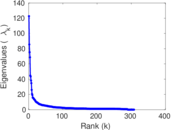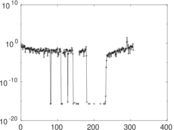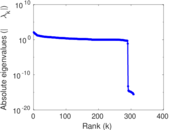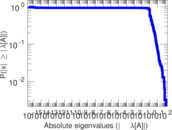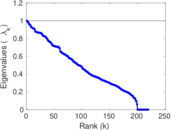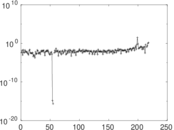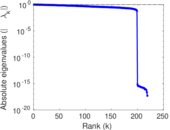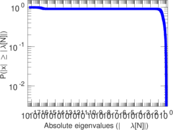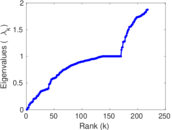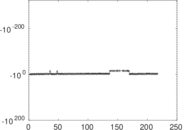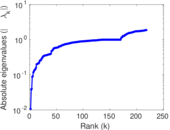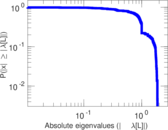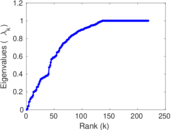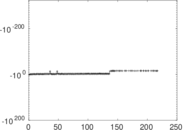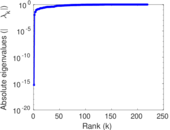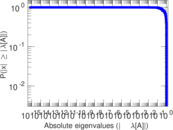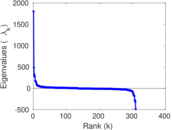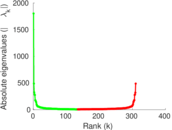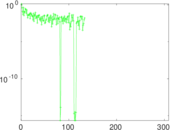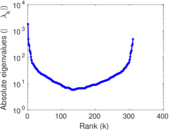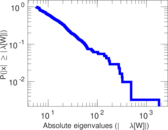# Wikiquote edits (et)

This is the bipartite edit network of the Estonian Wikiquote. It contains users and pages from the Estonian Wikiquote, connected by edit events. Each edge represents an edit. The dataset includes the timestamp of each edit.

 Code `qet` Internal name `edit-etwikiquote` Name Wikiquote edits (et) Data source http://dumps.wikimedia.org/ AvailabilityDataset is available for download Consistency checkDataset passed all tests Category Authorship network Dataset timestamp 2017-10-20 Node meaning User, article Edge meaning Edit Network formatBipartite, undirected Edge typeUnweighted, multiple edges Temporal dataEdges are annotated with timestamps

## Statistics

 Size n = 1,822 Left size n1 = 310 Right size n2 = 1,512 Volume m = 7,681 Unique edge count m̿ = 4,474 Wedge count s = 387,593 Claw count z = 35,614,684 Cross count x = 2,937,104,996 Square count q = 276,815 4-Tour count T4 = 3,779,604 Maximum degree dmax = 995 Maximum left degree d1max = 995 Maximum right degree d2max = 150 Average degree d = 8.431 39 Average left degree d1 = 24.777 4 Average right degree d2 = 5.080 03 Fill p = 0.009 545 14 Average edge multiplicity m̃ = 1.716 81 Size of LCC N = 1,559 Diameter δ = 14 50-Percentile effective diameter δ0.5 = 3.346 95 90-Percentile effective diameter δ0.9 = 5.221 43 Median distance δM = 4 Mean distance δm = 3.810 22 Gini coefficient G = 0.758 852 Balanced inequality ratio P = 0.203 945 Left balanced inequality ratio P1 = 0.119 516 Right balanced inequality ratio P2 = 0.265 070 Relative edge distribution entropy Her = 0.817 008 Power law exponent γ = 2.332 43 Tail power law exponent γt = 1.831 00 Tail power law exponent with p γ3 = 1.831 00 p-value p = 0.000 00 Left tail power law exponent with p γ3,1 = 1.671 00 Left p-value p1 = 0.165 000 Right tail power law exponent with p γ3,2 = 7.211 00 Right p-value p2 = 0.343 000 Degree assortativity ρ = −0.046 074 7 Degree assortativity p-value pρ = 0.002 051 88 Spectral norm α = 122.656 Algebraic connectivity a = 0.010 700 7 Spectral separation |λ1[A] / λ2[A]| = 1.434 07 Controllability C = 1,226 Relative controllability Cr = 0.678 848

## Plots

### Fruchterman–Reingold graph drawing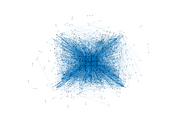### Degree distribution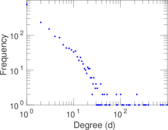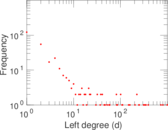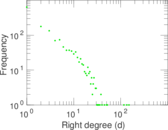### Cumulative degree distribution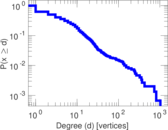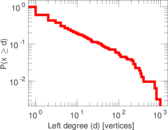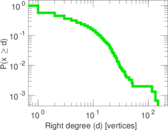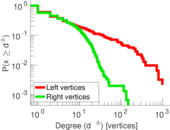### Lorenz curve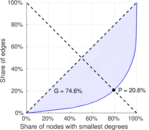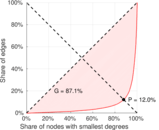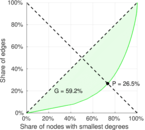### Spectral distribution of the adjacency matrix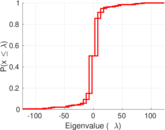### Spectral distribution of the normalized adjacency matrix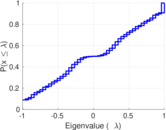### Spectral distribution of the Laplacian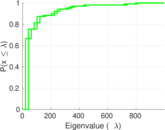### Spectral graph drawing based on the adjacency matrix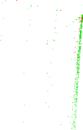### Spectral graph drawing based on the Laplacian### Spectral graph drawing based on the normalized adjacency matrix### Degree assortativity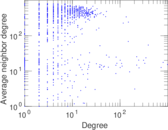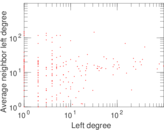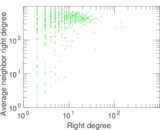### Zipf plot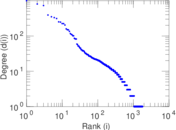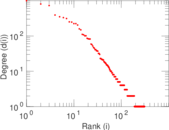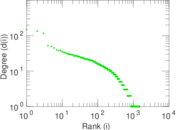### Hop distribution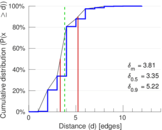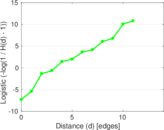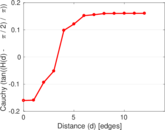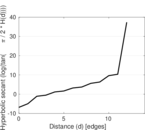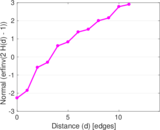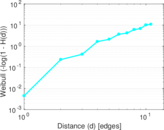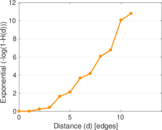### Double Laplacian graph drawing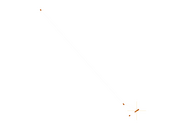### Delaunay graph drawing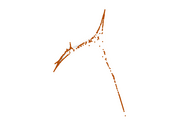### Edge weight/multiplicity distribution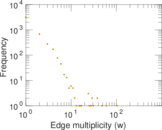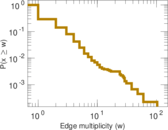### Temporal distribution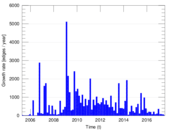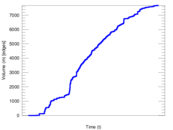### Temporal hop distribution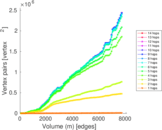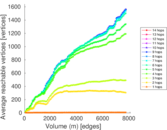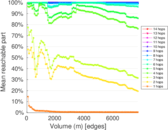### Diameter/density evolution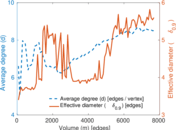### Matrix decompositions plots